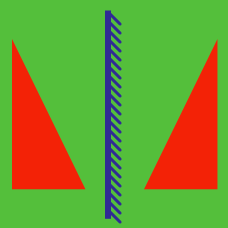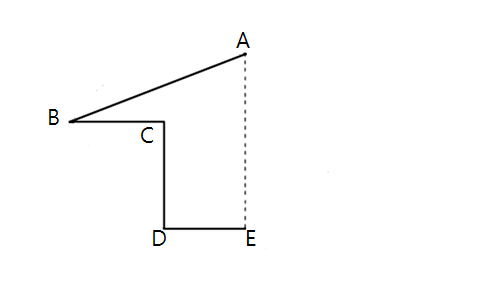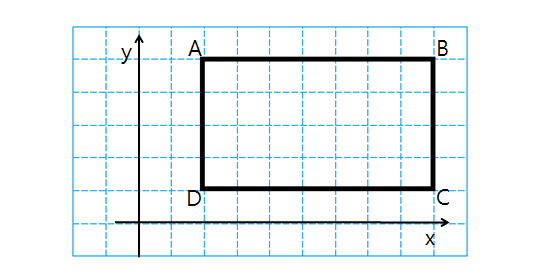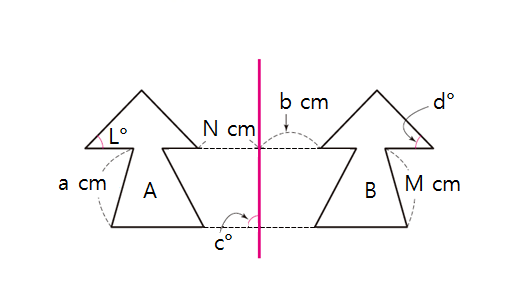Geometry

# ReflectionThe lengths of the lines in the above figure (a half house) are as follows: $\lvert \overline{AB} \rvert = 9, \lvert \overline{BC} \rvert = 3,$$\lvert \overline{CD} \rvert = 3, \lvert \overline{DE} \rvert = 3.$ If the shape is flipped across the line segment $\overline{AE}$ to complete a house, what will be the perimeter of the house?

The reflection of the circle $x^2+y^2-20x-20y+196=0$ in the origin $(0,0)\$ is $\ x^2+y^2+ax+by+c=0.$ What is $a+b+c$?

The point $A=(-4,5)$ is reflected in the line $y=x.$ What are the coordinates of the resulting image?If rectangle $ABCD$ in the above graph is reflected in the line $y = -1,$ what is the image of point $D?$ The side length of each square in the graph paper is $1.$In the above diagram, shape $B$ on the right is the reflection of shape $A$ in the red line. Given the following numbers: $L = 48, \quad N = 3, \quad M = 6$ in the diagram, what is the value of $a - b + c - d?$

×